# Kruskals Minimum Spanning Tree Algorithm

What is Minimum Spanning Tree?
Given a connected and undirected graph, a spanning tree of that graph is a subgraph that is a tree and connects all the vertices together. A single graph can have many different spanning trees. A minimum spanning tree (MST) or minimum weight spanning tree for a weighted, connected and undirected graph is a spanning tree with weight less than or equal to the weight of every other spanning tree. The weight of a spanning tree is the sum of weights given to each edge of the spanning tree.
How many edges does a minimum spanning tree has?
A minimum spanning tree has (V-1) edges where V is the number of vertices in the given graph.

Below are the steps for finding MST using Kruskal%u2019s algorithm

1. Sort all the edges in non-decreasing order of their weight.
2. Pick the smallest edge. Check if it forms a cycle with the spanning tree formed so far. If cycle is not formed, include this edge. Else, discard it.
3. Repeat step#2 until there are (V-1) edges in the spanning tree.

The step#2 uses Union-Find algorithm to detect cycle.

The algorithm is a Greedy Algorithm. The Greedy Choice is to pick the smallest weight edge that does not cause a cycle in the MST constructed so far. Let us understand it with an example: Consider the below input graph.The graph contains 9 vertices and 14 edges. So, the minimum spanning tree formed will be having (9 - 1) = 8 edges.

`After sorting:Weight   Src    Dest1         7      62         8      22         6      54         0      14         2      56         8      67         2      37         7      88         0      78         1      29         3      410        5      411        1      714        3      5`

Now pick all edges one by one from sorted list of edges
1. Pick edge 7-6: No cycle is formed, include it.2. Pick edge 8-2: No cycle is formed, include it.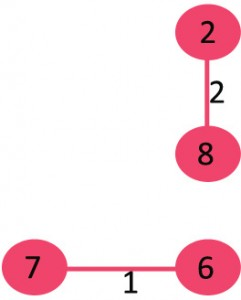3. Pick edge 6-5: No cycle is formed, include it.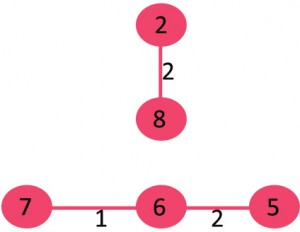4. Pick edge 0-1: No cycle is formed, include it.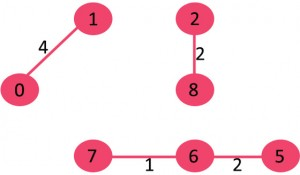5. Pick edge 2-5: No cycle is formed, include it.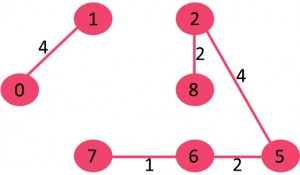6. Pick edge 8-6: Since including this edge results in cycle, discard it.
7. Pick edge 2-3: No cycle is formed, include it.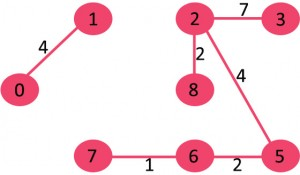8. Pick edge 7-8: Since including this edge results in cycle, discard it.
9. Pick edge 0-7: No cycle is formed, include it.10. Pick edge 1-2: Since including this edge results in cycle, discard it.
11. Pick edge 3-4: No cycle is formed, include it.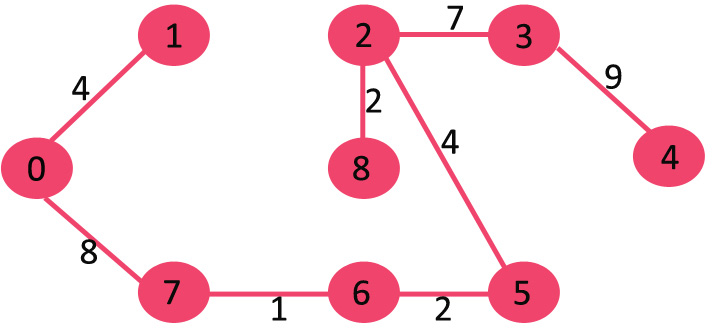Since the number of edges included equals (V- 1), the algorithm stops here

// C++ program for Kruskal's algorithm
// to find Minimum Spanning Tree of a
// given connected, undirected and weighted
// graph
#include <bits/stdc++.h>
using namespace std;

// a structure to represent a
// weighted edge in graph
class Edge {
public:
int src, dest, weight;
};

// a structure to represent a connected,
// undirected and weighted graph
class Graph {
public:

// V-> Number of vertices, E-> Number of edges
int V, E;

// graph is represented as an array of edges.
// Since the graph is undirected, the edge
// from src to dest is also edge from dest
// to src. Both are counted as 1 edge here.
Edge* edge;
};

// Creates a graph with V vertices and E edges
Graph* createGraph(int V, int E)
{
Graph* graph = new Graph;
graph->V = V;
graph->E = E;

graph->edge = new Edge[E];

return graph;
}

// A structure to represent a subset for union-find
class subset {
public:
int parent;
int rank;
};

// A utility function to find set of an element i
// (uses path compression technique)
int find(subset subsets[], int i)
{
// find root and make root as parent of i
// (path compression)
if (subsets[i].parent != i)
subsets[i].parent
= find(subsets, subsets[i].parent);

return subsets[i].parent;
}

// A function that does union of two sets of x and y
// (uses union by rank)
void Union(subset subsets[], int x, int y)
{
int xroot = find(subsets, x);
int yroot = find(subsets, y);

// Attach smaller rank tree under root of high
// rank tree (Union by Rank)
if (subsets[xroot].rank < subsets[yroot].rank)
subsets[xroot].parent = yroot;
else if (subsets[xroot].rank > subsets[yroot].rank)
subsets[yroot].parent = xroot;

// If ranks are same, then make one as root and
// increment its rank by one
else {
subsets[yroot].parent = xroot;
subsets[xroot].rank++;
}
}

// Compare two edges according to their weights.
// Used in qsort() for sorting an array of edges
int myComp(const void* a, const void* b)
{
Edge* a1 = (Edge*)a;
Edge* b1 = (Edge*)b;
return a1->weight > b1->weight;
}

// The main function to construct MST using Kruskal's
// algorithm
void KruskalMST(Graph* graph)
{
int V = graph->V;
Edge result[V]; // Tnis will store the resultant MST
int e = 0; // An index variable, used for result[]
int i = 0; // An index variable, used for sorted edges

// Step 1: Sort all the edges in non-decreasing
// order of their weight. If we are not allowed to
// change the given graph, we can create a copy of
// array of edges
qsort(graph->edge, graph->E, sizeof(graph->edge),
myComp);

// Allocate memory for creating V ssubsets
subset* subsets = new subset[(V * sizeof(subset))];

// Create V subsets with single elements
for (int v = 0; v < V; ++v)
{
subsets[v].parent = v;
subsets[v].rank = 0;
}

// Number of edges to be taken is equal to V-1
while (e < V - 1 && i < graph->E)
{
// Step 2: Pick the smallest edge. And increment
// the index for next iteration
Edge next_edge = graph->edge[i++];

int x = find(subsets, next_edge.src);
int y = find(subsets, next_edge.dest);

// If including this edge does't cause cycle,
// include it in result and increment the index
// of result for next edge
if (x != y) {
result[e++] = next_edge;
Union(subsets, x, y);
}
// Else discard the next_edge
}

// print the contents of result[] to display the
// built MST
cout << "Following are the edges in the constructed "
"MST\n";
int minimumCost = 0;
for (i = 0; i < e; ++i)
{
cout << result[i].src << " -- " << result[i].dest
<< " == " << result[i].weight << endl;
minimumCost = minimumCost + result[i].weight;
}
// return;
cout << "Minimum Cost Spanning Tree: " << minimumCost
<< endl;
}

// Driver code
int main()
{
/* Let us create following weighted graph
10
0--------1
| |
6| 5 |15
| |
2--------3
4 */
int V = 4; // Number of vertices in graph
int E = 5; // Number of edges in graph
Graph* graph = createGraph(V, E);

// add edge 0-1
graph->edge.src = 0;
graph->edge.dest = 1;
graph->edge.weight = 10;

// add edge 0-2
graph->edge.src = 0;
graph->edge.dest = 2;
graph->edge.weight = 6;

// add edge 0-3
graph->edge.src = 0;
graph->edge.dest = 3;
graph->edge.weight = 5;

// add edge 1-3
graph->edge.src = 1;
graph->edge.dest = 3;
graph->edge.weight = 15;

// add edge 2-3
graph->edge.src = 2;
graph->edge.dest = 3;
graph->edge.weight = 4;

// Function call
KruskalMST(graph);

return 0;
}

Output
`Following are the edges in the constructed MST2 -- 3 == 40 -- 3 == 50 -- 1 == 10Minimum Cost Spanning Tree: 19`

Time Complexity: O(ElogE) or O(ElogV). Sorting of edges takes O(ELogE) time. After sorting, we iterate through all edges and apply find-union algorithm. The find and union operations can take atmost O(LogV) time. So overall complexity is O(ELogE + ELogV) time. The value of E can be atmost O(V2), so O(LogV) are O(LogE) same. Therefore, overall time complexity is O(ElogE) or O(ElogV)#### More Articles of M Mounika:

Name Views Likes
C++ Segmented Sieve (Print Primes In a Range) 162 0
C++ Sieve Of Erastosthenes 135 0
C++ Gold Mine Problem 295 0
C++ Merge K Sorted Arrays 116 0
C++ K Centers Problem 239 0
C++ Find Nth Catalan Number 311 0
C++ Inplace Rotate square matrix by 90 degrees 285 0
C++ Find Non Repeating Elements in Array 86 0
C++ Merge Two Binary Trees 120 0
C++ Sum of Numbers From Root To Leaf Paths 89 0
C++ Meta Strings 91 0
C++ Flood Fill Algorithm 402 0
C++ smallest substring with maximum distinct characters 199 0
C++ Smallest window with all characters in string 93 0
C++ Minimum Removal of Characters from string to make its permutation as palindrome 86 0
C++ Minimum characters added at front of string in palindrome conversion 69 0
C++ Number of Bracket Reversals needed to make expression Balanced 72 0
C++ String to Palindrome with Append Function 83 0
C++ WildCard pattern matching 75 0
C++ Anagram substring Search 72 0
C++ Manachars Algorithm 74 0
C++ Search String in Grid 83 0
C++ String Matching(Z Algorithm) 67 0
C++ String Matching(Naive Algorithm) 113 0
C++ String Matching(KMP Algorithm) 140 0
C++ Remove Duplicates From String 110 0
C++ Basics of String Manipulation 85 1
C++ Disjoint Data Structure Cycle Detection 86 0
C++ Problem On Disjoint Data Structures 94 0
C++ Disjoint Data Structures Part3 78 0
Disjoint Data Structures Part2 90 0
Disjoint Data Structures 93 1
C++ Segment Trees 321 2
C++ Trie Cost of Data 290 1
C++ Trie Datastructure 278 1
C++ Greedy Approach Minimum number of coins 525 0
C++ Greedy Approach Maximum height Pyramid 328 1
C++ Greedy Approach String lexicographically largest subsequence 246 0
C++ Greedy Approach Lexicographically largest subsequence 364 0
C++ Greedy Approach Prims MST 398 1
C++ Greedy Approach Krushkals MST 458 1
C++ Greedy Approach N-array maximum sum 333 1
C++ Greedy Approach Policemen Catch Thieves 563 1
C++ Greedy Approach Maximum product Subset 546 1
C++ Greedy Approach Minimum Product Subset 348 1
C++ Greedy Approach Fractional Knapsack 737 1
C++ Greedy Approach-Activity Selection Problem 745 1
C++ Greedy Approach-Egyptian Fractions 639 0
C++ Greedy Approach-Huffman Codes 1031 1
C++ Introduction to Greedy Approach 955 2